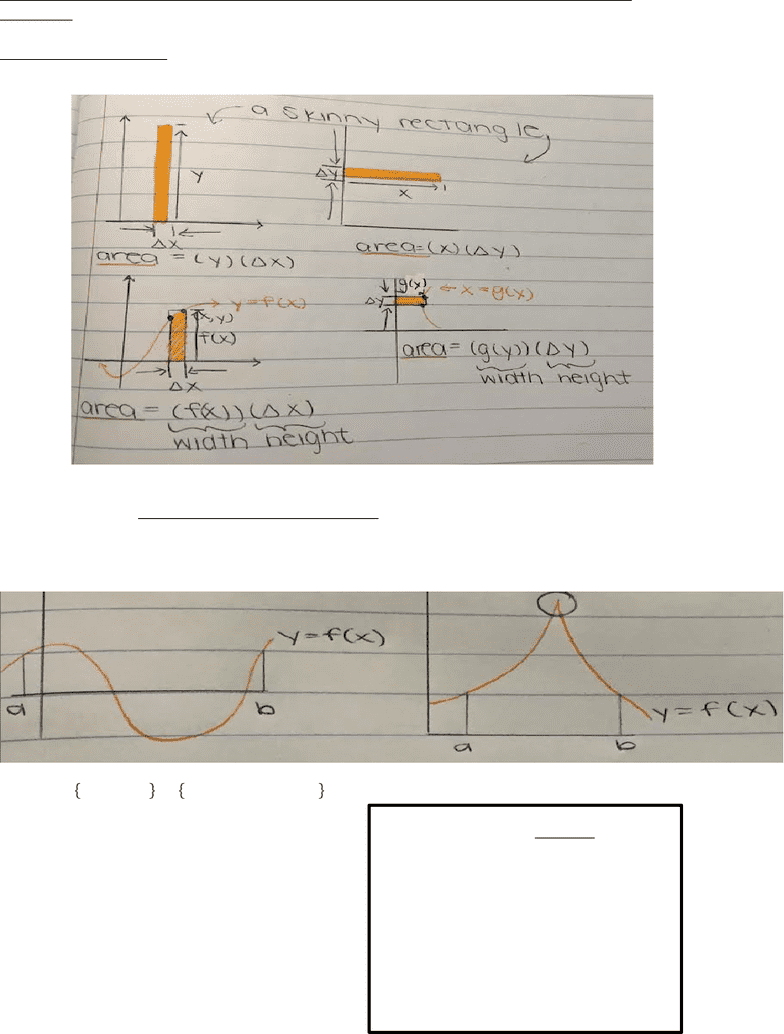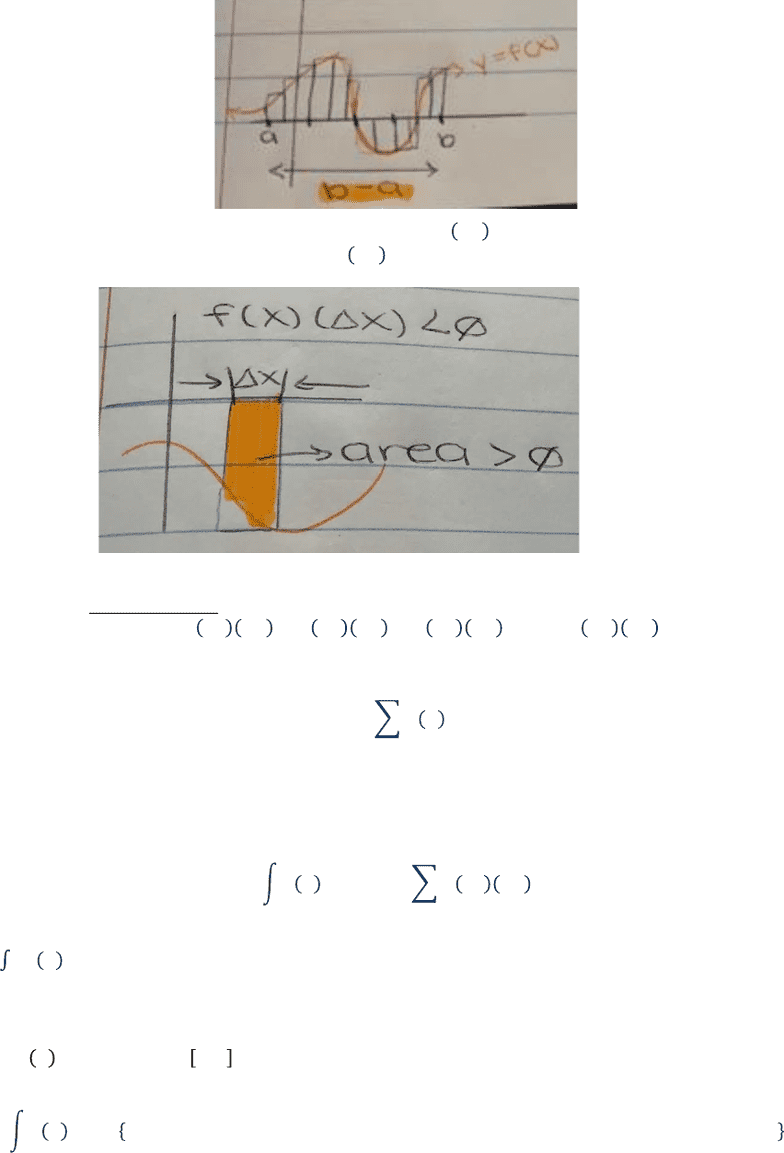# MATA32 Lecture 21: The Definite Integral and the Fundamental Theorem of Calculus

53 views5 pages
School
Department
CourseMATA32 Lecture 21: The Definite Integral and the Fundamental Theorem of
Calculus
The Definite Integral
We’ll see that the definition of the definite integral is based on these simple ideas
Begin with a given interval [a,b] (a<b) and a continuous MATA32 function y= f(x) where
𝑥[𝑎,𝑏]
Let 𝑛𝜖!=1,2,3,4=𝑛𝑎𝑡𝑢𝑟𝑎𝑙!𝑛𝑢𝑚𝑏𝑒𝑟𝑠
𝐿𝑒𝑡!𝑥=
(
𝑏𝑎
)
𝑛
=Sub-interval width
As ‘n’ gets larger and larger 𝑥 gets
smaller and smaller
lim
!!
𝑥=0
Unlock document

This preview shows pages 1-2 of the document.
Unlock all 5 pages and 3 million more documents.Right endpoint of the kth sub-interval is 𝑥!=𝑎+𝑘𝑥=𝑎,𝑤𝑒𝑟𝑒!𝑘=0!
𝑥!=𝑎+𝑛𝑥=𝑏,𝑤𝑒𝑟𝑒!𝑘=𝑛
In the nth Riemann Sum is defined as follows:
𝑆𝑛 =𝑓𝑥1𝑥+𝑓𝑥2𝑥+𝑓𝑥3𝑥++𝑓𝑥𝑛 𝑥
In the Summation Notation (Sigma Notation)
𝑆𝑛 =𝑓𝑘𝑥
!
!!!
** ‘k’ must start at 1
Definition: The definite integral of y=f(x) from ‘a’ to ‘b’ is:
𝑓𝑥𝑑𝑥
!
!
=lim
!!𝑓𝑥!𝑥
!
!!!
𝑓𝑥𝑑𝑥 =#
!
! is a special real number that is the limit of a certain kind of sum
Special, Important Case:
If 𝑓𝑥0 when 𝑥𝑎,𝑏 then,
𝑓𝑥𝑑𝑥
!
!
=!𝑡𝑒!𝑎𝑟𝑒𝑎!𝑜𝑓!𝑡𝑒!𝑟𝑒𝑔𝑖𝑜𝑛!𝑢𝑛𝑑𝑒𝑟!𝑡𝑒!𝑐𝑢𝑟𝑣𝑒,𝑎𝑏𝑜𝑣𝑒!𝑥𝑎𝑥𝑖𝑠!𝑤𝑒𝑟𝑒!𝑎𝑥𝑏
Unlock document

This preview shows pages 1-2 of the document.
Unlock all 5 pages and 3 million more documents.

# Get access

\$10 USD/m
Billed \$120 USD annually
Homework Help
Class Notes
Textbook Notes